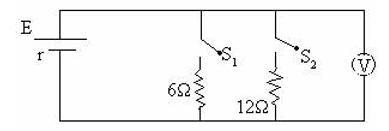voltmeter
Question

# In the circuit when switch ${\mathrm{S}}_{1}$ is closed and ${\mathrm{S}}_{2}$ is open, the ideal voltmeter shows a reading 18V. When switch ${\mathrm{S}}_{2}$ is closed and ${\mathrm{S}}_{1}$ is open, the reading of voltmeter 24V. When ${\mathrm{S}}_{1}$ and ${\mathrm{S}}_{2}$ both are closed the voltmeter reading will beModerate
Solution

## When ${\mathrm{S}}_{1}$  is closed  $\left(\frac{\mathrm{E}}{6+\mathrm{r}}\right)6=18\to \left(1\right)$When only  ${\mathrm{S}}_{2}$ closed $\left(\frac{\mathrm{E}}{12+\mathrm{r}}\right)12=24\to \left(2\right)$Dividing (1) and (2)  $\left(\frac{12+\mathrm{r}}{6+\mathrm{r}}\right)\frac{1}{2}=\frac{3}{4}$ $24+2\mathrm{r}=18+3\mathrm{r}$ $\mathrm{r}=6\mathrm{\Omega }$When both are closed $\frac{6×12}{18}=4\mathrm{\Omega }$$\frac{\mathrm{V}}{18}=\frac{\left(\frac{\mathrm{E}}{4+\mathrm{r}}\right)4}{\left(\frac{\mathrm{E}}{6+\mathrm{r}}\right)6}\frac{\mathrm{V}}{6}=\frac{2\left(6+6\right)}{\left(4+6\right)}$$\mathrm{V}=\frac{12×12}{10}=14.4\mathrm{V}$

Get Instant Solutions